# Excel Logical Function – AND, OR FunctionHello Everyone, In this tutorial I will be talking about the Basics of 2 Logical Functions which are And Formula & OR Formula and we will see that in how many ways you can ask a question in Excel.

So let me help you with the help of 2 examples for each formula so that you all can get to know what the formula is all about and how it works50% OFF on Excel Course

• Get 2-Year Access with unlimited views of 220+ videos
• Use Coupon code - NAV50OFF & Start learning

Example 1: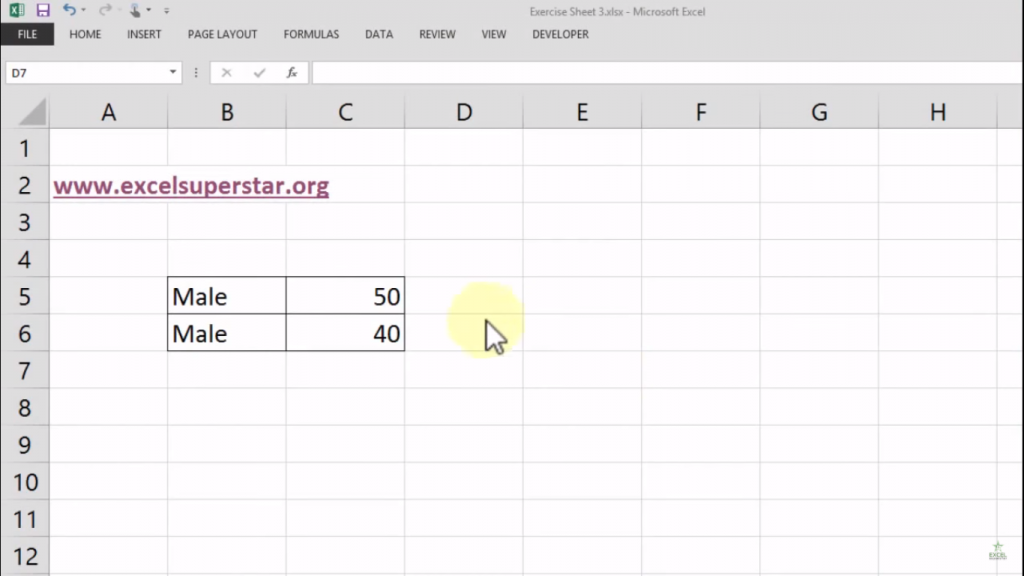In this example, if I want to ask 2 question which is:

1. Is the person’s name is male or not?

2.Is the person’s age is above 45?

So to get the answer we have to apply a formula which is called AND Formula. Let us see how to apply this formula to get the answer

1. Write =AND(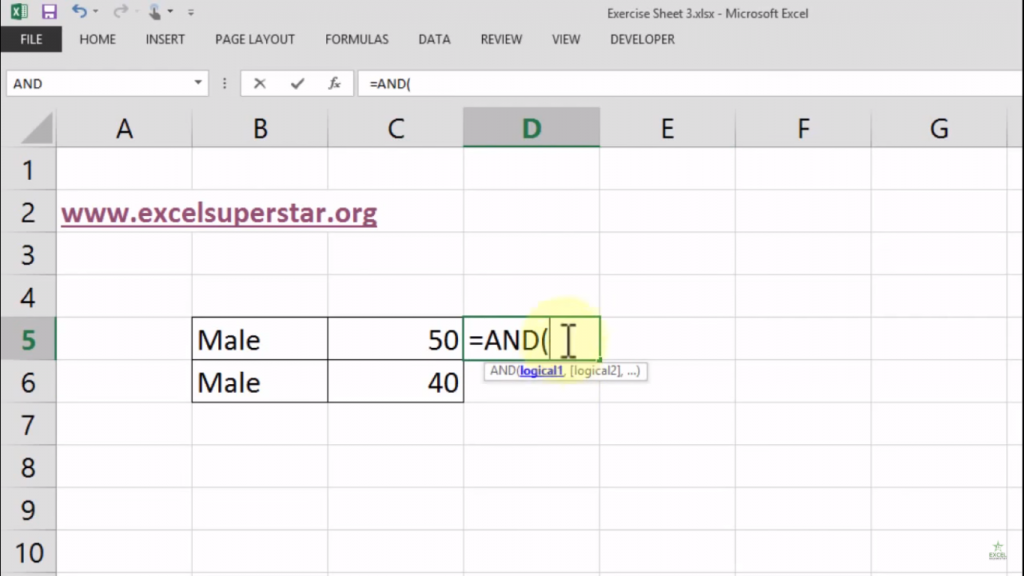Now here it has 2 logical parameters named Logical 1, Logical 2, . So the first logical question will be Is the person’s name is male or not? To get the answer what we will do is

2. Choose the Male cell so the formula will be =AND(B5″MALE”,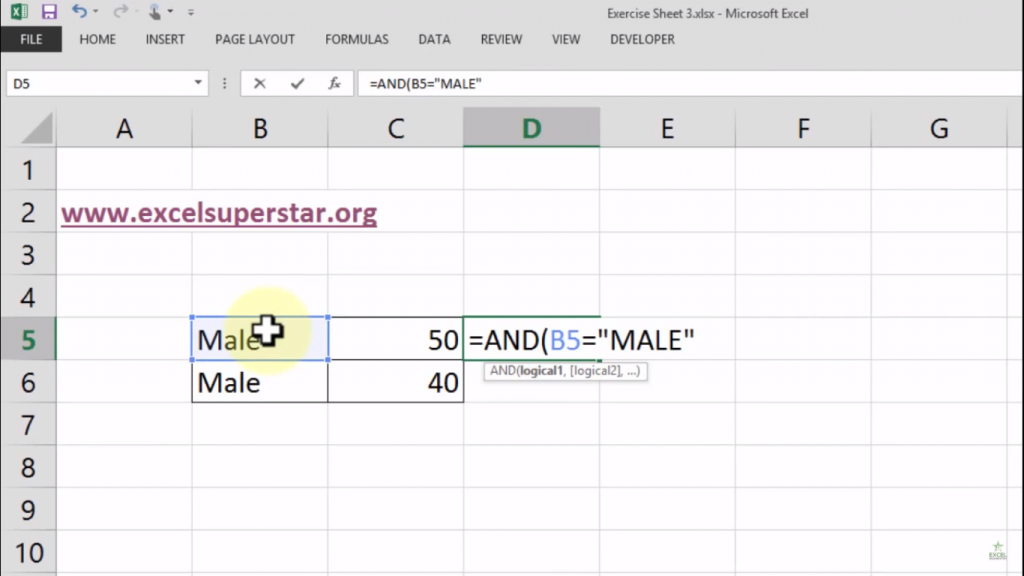The second logical question will be TRUE or FALSE which means the answer should be in TRUE or FALSE only

3. Choose the cell named as “50” and write >=45 so the formula will be =AND(B5″MALE”, C5>=45)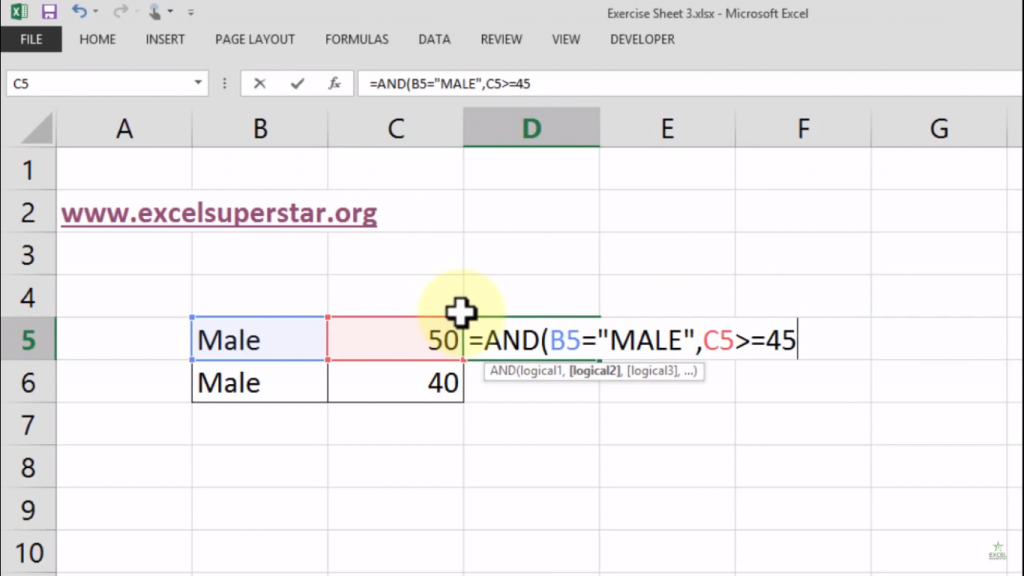4. Lastly, press Enter

5. But here it is different which means: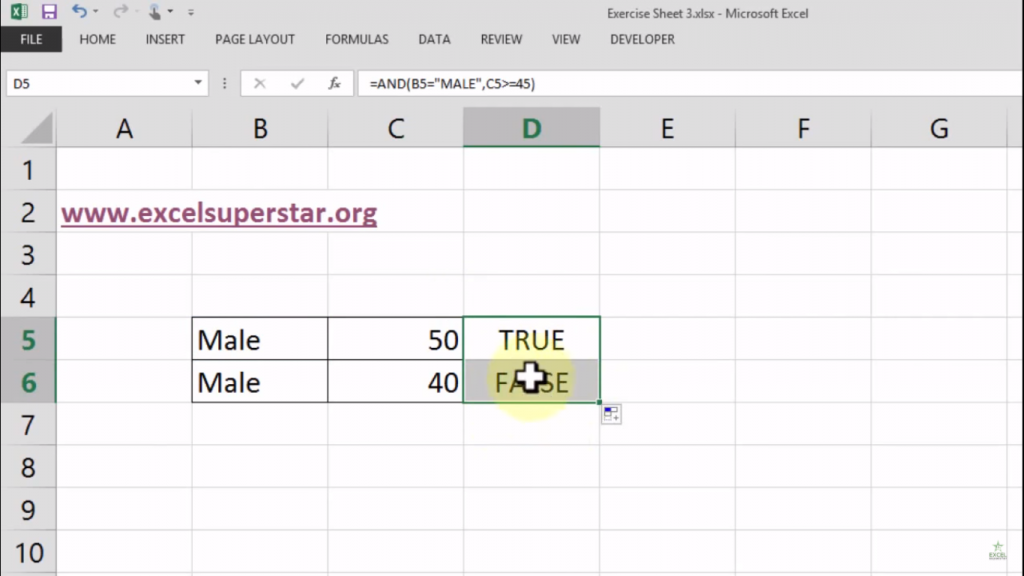1. In the first case it is TRUE because it answers both the questions

2. In the second case it is FALSE because the person is male but the age of a person is below 45

Example 2:

In this example, if I want to ask 2 question which is:

1. Is the person’s name is male or not?

2. Is the person’s age is 40 and above?

So to get the answer we have to apply a formula which is called OR Formula. Let us see how to apply this formula to get the answer

1. Write =OR(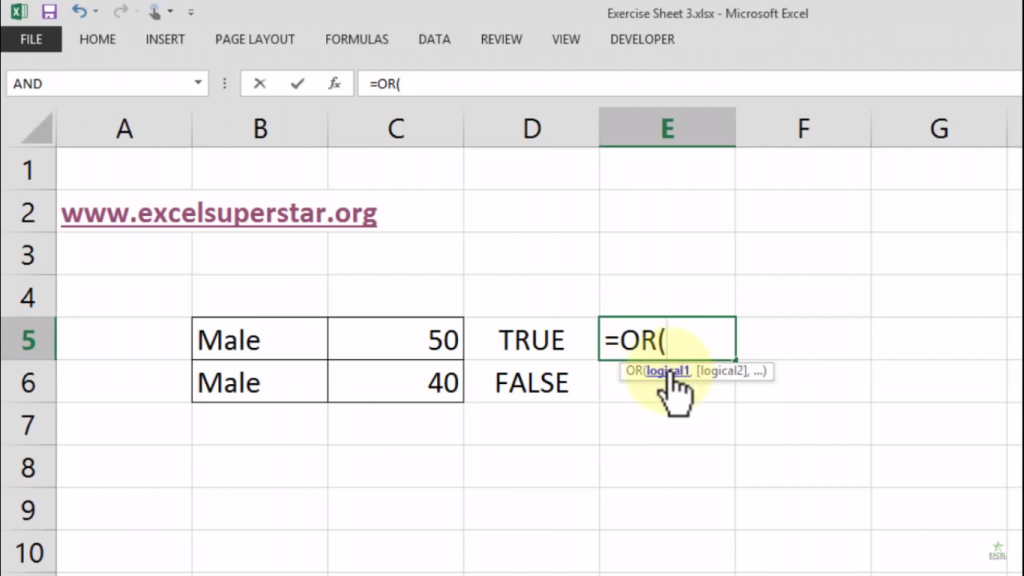Now here it has 2 logical parameters named Logical 1, Logical 2, . So the first logical question will be Is the person’s name is male or not? To get the answer what we will do is

2. Choose the Male cell so the formula will be =OR(B5″Male”,

The second logical question will be TRUE or FALSE which means the answer should be in TRUE or FALSE only

3. Choose the cell named as “50” and write >=45 so the formula will be =AND(B5″MALE”, C5>=45)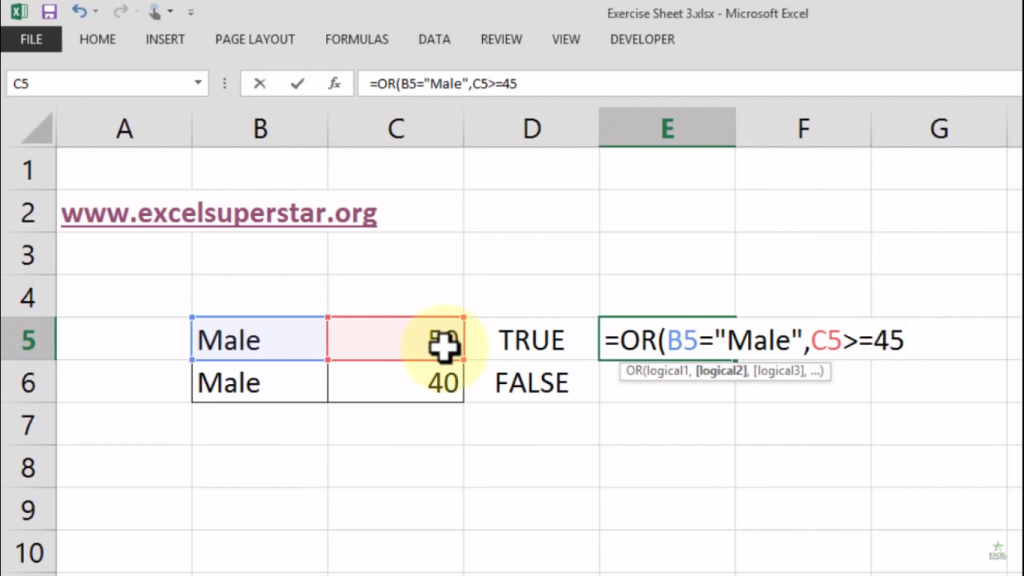4. Press Enter

5. So here above-given questions give me one answer which is TRUE in both the cases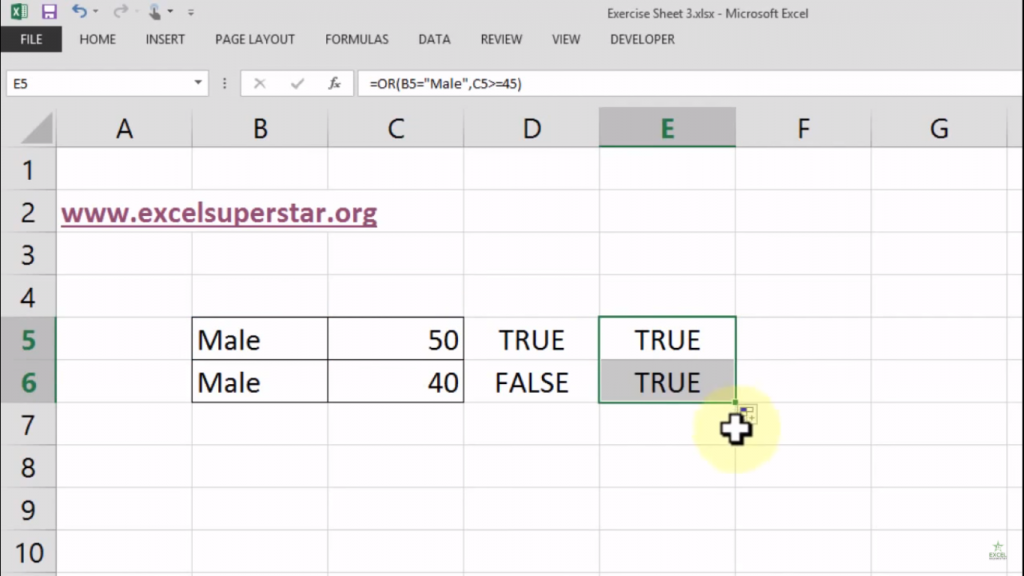Conclusion:

So practice these basics of AND Formula and OR Formula in Excel so that you can do your work of question and answer easily in your Excel. Also if you have any doubts then do comment below I will be glad to help you out.